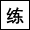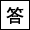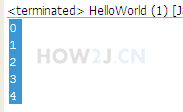控制流程系列教材 （二）- java的switch语句 控制流程系列教材 （四）- java的for循环

while和do-while循环语句4分25秒

while

do while## 条件为true时 重复执行public class HelloWorld { public static void main(String[] args) { //打印0到4 int i = 0; while(i<5){ System.out.println(i); i++; } } }
```public class HelloWorld {
public static void main(String[] args) {

//打印0到4
int i = 0;
while(i<5){
System.out.println(i);
i++;
}
}
}
```

## 条件为true时 重复执行，至少会执行一次

do{
} while 循环public class HelloWorld { public static void main(String[] args) { //打印0到4 //与while的区别是，无论是否成立，先执行一次，再进行判断 int i = 0; do{ System.out.println(i); i++; } while(i<5); } }
```public class HelloWorld {
public static void main(String[] args) {

//打印0到4
//与while的区别是，无论是否成立，先执行一次，再进行判断
int i = 0;
do{
System.out.println(i);
i++;
} while(i<5);

}
}
```

## 练习-阶乘

姿势不对,事倍功半! 点击查看做练习的正确姿势

N的阶乘等于 N* (N-1) * (N-2) * ... * 1## 答案-阶乘4分44秒 本视频采用html5方式播放，如无法正常播放，请将浏览器升级至最新版本，推荐火狐，chrome，360浏览器。 如果装有迅雷，播放视频呈现直接下载状态，请调整 迅雷系统设置-基本设置-启动-监视全部浏览器 (去掉这个选项)。 chrome 的 视频下载插件会影响播放，如 IDM 等，请关闭或者切换其他浏览器

import java.util.Scanner; public class HelloWorld { public static void main(String[] args) { Scanner s = new Scanner(System.in); System.out.println("请输入一个整数："); int n = s.nextInt(); int fac = 1; while(n>=1){ fac *=n; n--; } System.out.println("阶乘是:" + fac); } }
```import java.util.Scanner;

public class HelloWorld {
public static void main(String[] args) {
Scanner s = new Scanner(System.in);
System.out.println("请输入一个整数：");
int n = s.nextInt();
int fac = 1;
while(n>=1){
fac *=n;
n--;
}
System.out.println("阶乘是:" + fac);
}
}
```

 控制流程系列教材 （二）- java的switch语句 控制流程系列教材 （四）- java的for循环

HOW2J公众号，关注后实时获知最新的教程和优惠活动，谢谢。2020-10-15 为什么输出是负数？哪里有问题？
 ballrice 关于 JAVA 基础-控制流程-while 的提问 计算结果是负数。是定义的类型不对吗？ ```import java.util.Scanner; public class jiecheng { public static void main(String[] args) { Scanner s = new Scanner(System.in); System.out.println("请输入一个整数"); int N = s.nextInt(); int a = 1; do{ N*= a; a++; } while(a < N); System.out.println("阶乘是："+N); } }```

8 个答案

2330695344

public static void main(String[]args) { Scanner sc=new Scanner(System.in); System.out.println("请输入一个正整数"); int a=sc.nextInt(); int sum=1; while(a>1) { sum*=a; a--; }System.out.println("阶乘是"+sum); }

hmqk

public static void main(String[] args) { Scanner sc = new Scanner(System.in); System.out.println("请输入一个整数："); int a = sc.nextInt(); int b=a; int jc =1; while(a>=1) { jc *=a; a--; } System.out.println(b+"的阶乘是："+jc); }

nameone

N*= a; N在变化

SKY_b

2020-10-14 打卡
 cbh 关于 JAVA 基础-控制流程-while 的提问 public static int df(int i){ if (i == 1){ return 1; } return i * df(i - 1); }

9 个答案

valecalida

2330695344

public static void jiecheng(){ Scanner scanner=new Scanner(System.in); System.out.println("请输入一个整数计算阶乘:"); int n=scanner.nextInt(); int sum=1; while(n>1){ sum*=n; n--; } System.out.println("该数的阶乘为："+sum); } public static void main(String[] args){ jiecheng(); }

package controllerliucheng; import java.util.Scanner; public class jiecheng { public static void main(String[] args) { Scanner scanner=new Scanner(System.in); int i=scanner.nextInt(); int n=1; int sum=1; int a=i; while(n<=i){ sum=sum*i; i--; } System.out.println(a+"的阶乘为："+sum); } }

bobai

import java.util.Scanner; public class Demo1 { public static void main(String[] args) { Scanner sc = new Scanner(System.in); System.out.println("请输入一个整数："); int i = sc.nextInt(); int jc= 1; while(i>=1){ jc*=i; i--; } System.out.println(i+"阶乘为："+jc); } }

hmqk

public static void main(String[] args) { Scanner sc = new Scanner(System.in); System.out.println("请输入一个整数："); int a = sc.nextInt(); int b=a; int jc =1; while(a>=1) { jc *=a; a--; } System.out.println(b+"的阶乘是："+jc); }

babab

public static void main(String[] args) { Scanner sc = new Scanner(System.in); int num = 0; System.out.println("请输入您要计算的阶乘："); num = sc.nextInt(); int sum = 1; int i = 1; while(i<=num){ sum *= i; i++; } System.out.println("阶乘为：" + sum); }

2020-10-13 阶乘问题
2020-09-10 請問哪裡有錯
2020-08-30 请问为什么输入100后阶乘显示等于0？